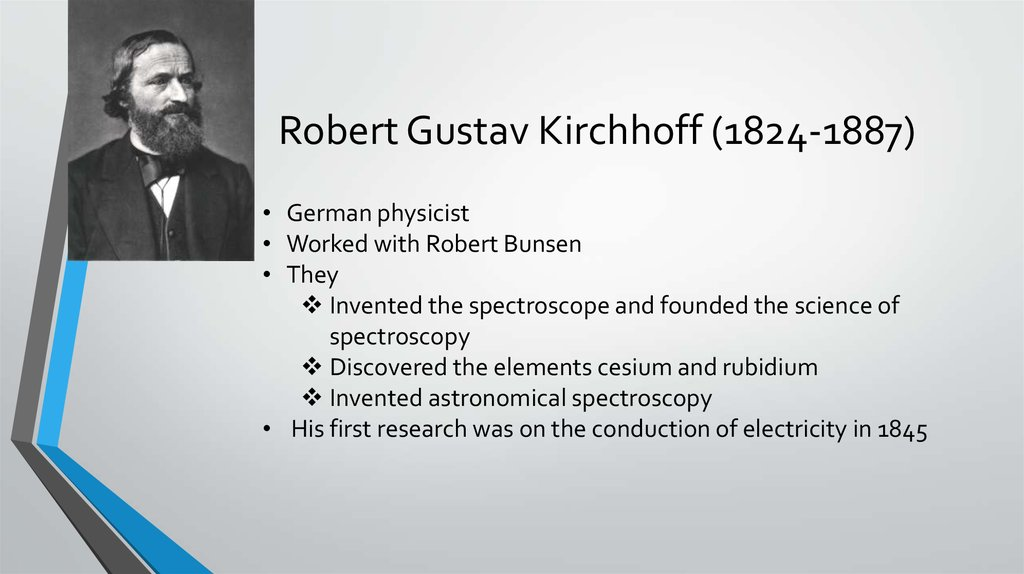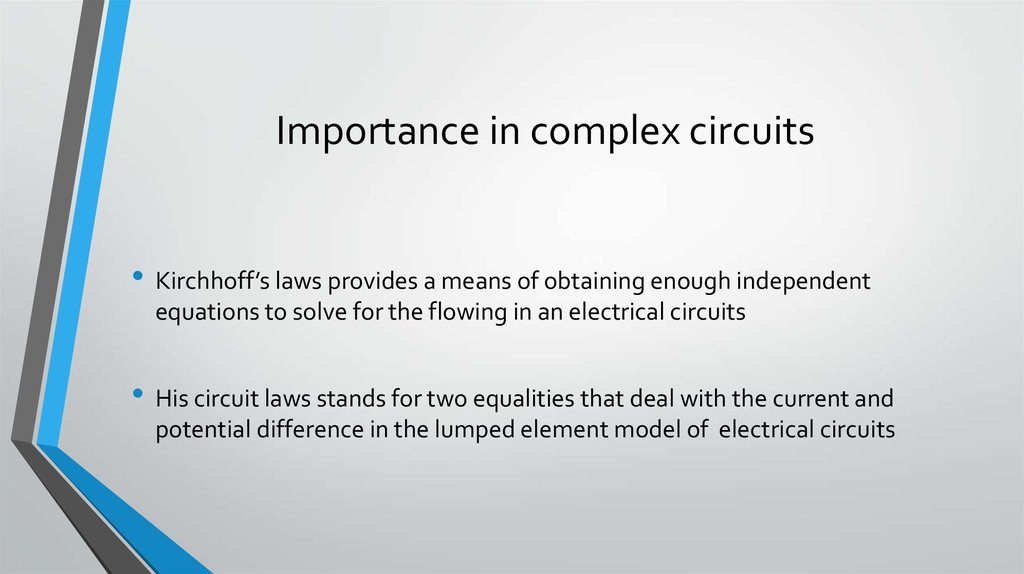# Kirchhoff’s Laws for circuits

## 2. Robert Gustav Kirchhoff (1824-1887)

• German physicist
• Worked with Robert Bunsen
• They
Invented the spectroscope and founded the science of
spectroscopy
Discovered the elements cesium and rubidium
Invented astronomical spectroscopy
• His first research was on the conduction of electricity in 1845

## 3. Importance in complex circuits

• Kirchhoff’s laws provides a means of obtaining enough independent
equations to solve for the flowing in an electrical circuits
• His circuit laws stands for two equalities that deal with the current and
potential difference in the lumped element model of electrical circuits

## 4. Kirchhoff's Circuit Law

• We saw in the Resistors tutorial that a single equivalent resistance, ( RT ) can be
found when two or more resistors are connected together in either series, parallel
or combinations of both, and that these circuits obey Ohm’s Law
• However, sometimes in complex circuits such as bridge or T networks, we can not
simply use Ohm’s Law alone to find the voltages or currents circulating within the
circuit. For these types of calculations we need certain rules which allow us to
obtain the circuit equations and for this we can use Kirchhoff's Circuit Law

## 5. Kirchhoff's First Law – The Current Law, (KCL)

• Kirchhoff's Current Law or KCL, states that the “total current or charge
entering a junction or node is exactly equal to the charge leaving the node as it
has no other place to go except to leave, as no charge is lost within the node“.
In other words the algebraic sum of ALL the currents entering and leaving a
node must be equal to zero, I(exiting) + I(entering) = 0. This idea by Kirchhoff is
commonly known as the Conservation of Charge.

## 6. Kirchhoff's Current Law

Algebraic sum of ALL the currents entering and leaving a
node must be equal to zero, I(exiting) + I(entering) = 0.
Here, the 3 currents entering the node, I1, I2, I3 are all positive
in value and the 2 currents leaving the node, I4 and I5 are
negative in value. Then this means we can also rewrite the
equation as;
I1 + I2 + I3 – I4 – I5 = 0
The term Node in an electrical circuit
generally refers to a connection or junction
of two or more current carrying paths or
elements such as cables and components.

## 7. Kirchhoff's Second Law – The Voltage Law, (KVL)

Kirchhoff's Voltage Law or KVL, states that “in any closed loop network, the total voltage around the
loop is equal to the sum of all the voltage drops within the same loop” which is also equal to zero. In
other words the algebraic sum of all voltages within the loop must be equal to zero. This idea by
Kirchhoff is known as the Conservation of Energy.
Starting at any point in the loop continue in the same direction noting the direction of all the voltage
drops, either positive or negative, and returning back to the same starting point. It is important to
maintain the same direction either clockwise or anti-clockwise or the final voltage sum will be equal to
zero. We can use Kirchhoff's voltage law when analyzing series circuits.
When analyzing either DC circuits or AC circuits using
Kirchhoff's Circuit Laws a number of definitions and
terminologies are used to describe the parts of the circuit
being analyzed such as: node, paths, branches, loops and
meshes. These terms are used frequently in circuit
analysis so it is important to understand them.

## 8. Common DC Circuit Theory Terms:

• Circuit – a circuit is a closed loop conducting path in which an electrical current flows.
• Path – a single line of connecting elements or sources.
• Node – a node is a junction, connection or terminal within a circuit were two or more circuit
elements are connected or joined together giving a connection point between two or more
branches. A node is indicated by a dot.
• Branch – a branch is a single or group of components such as resistors or a source which are
connected between two nodes.
• Loop – a loop is a simple closed path in a circuit in which no circuit element or node is encountered
more than once.
A Typical DC Circuit

## 9. Application of Kirchhoff's Circuit Laws

These two laws enable the Currents and Voltages in a circuit to be found, if, the circuit is said to be
“Analyzed”, and the basic procedure for using Kirchhoff's Circuit Laws is as follows:
1. Assume all voltages and resistances are given. ( If not label them V1, V2,… R1, R2, etc. )
2. Label each branch with a branch current. ( I1, I2, I3 etc. )
3. Find Kirchhoff's first law equations for each node. (KCL)
4. Find Kirchhoff's second law equations for each of the independent loops of the circuit. (KVL)
5. Use Linear simultaneous equations as required to find the unknown currents.

## 10. Conclusion

• By knowing and using Kirchhoff’s Laws we can simplify our calculations and
reduce more amount of mathematics in solving for circuits.